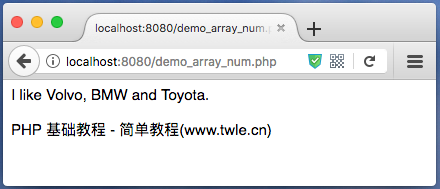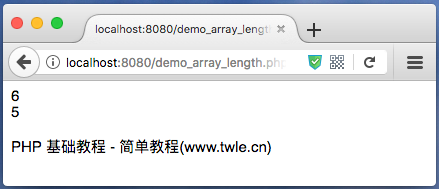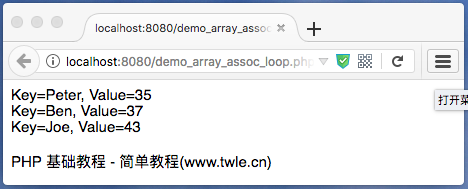PHP 数组 ( array )

范例

```<?php

\$cars=array("Volvo","BMW","Toyota");

echo "I like " . \$cars . ", " . \$cars . " and " . \$cars . ".","<br/>";

echo '<p>PHP 基础教程 - 简单教程(www.twle.cn)</p>';
```数组是什么？

```<?php
\$cars1 = "Volvo";
\$cars2 = "BMW";
\$cars3 = "Toyota";
```

PHP 语言创建数组

PHP 提供了 `array()` 函数用于创建数组

PHP 语言提供了三种类型的数组

1. 数值数组 ： 带有数字 ID 键的数组
2. 关联数组 ： 带有指定的键的数组，每个键关联一个值
3. 多维数组 ： 包含一个或多个数组的数组

PHP 数值数组

PHP 语言中创建数值数组有两种方法

1. 自动分配 id 键（id 键总是从 0 开始

```\$cars = array("Volvo","BMW","Toyota");
```
2. 人工分配 id 键

```\$cars = "Volvo";
\$cars = "BMW";
\$cars="Toyota";
```

范例

```<?php

\$cars=array("Volvo","BMW","Toyota");
echo "I like " . \$cars . ", " . \$cars . " and " . \$cars . ".","<br/>";

echo '<p>PHP 基础教程 - 简单教程(www.twle.cn)</p>';
```PHP 操作数组相关的函数

获取数组的长度 - count() 函数

PHP count() 函数用于返回数组的长度（元素的数量）

```<?php

\$age=array("11"=>"27","11a"=>"29","12a"=>"37","Bill"=>"35","Steve"=>"37","Peter"=>"43");
echo count(\$age),"<br/>";
unset(\$age["11"]);
echo count(\$age),"<br/>";
echo '<p>PHP 基础教程 - 简单教程(www.twle.cn)</p>';
```遍历数值数组

```<?php
\$cars=array("Volvo","BMW","Toyota");
\$arrlength=count(\$cars);

for(\$x=0;\$x<\$arrlength;\$x++)
{
echo \$cars[\$x];
echo "<br>";
}
echo '<p>PHP 基础教程 - 简单教程(www.twle.cn)</p>';
```PHP 关联数组

PHP 语言创建关联数组有两种方式

```<?php
\$age=array("Peter"=>"35","Ben"=>"37","Joe"=>"43");
```

```<?php

\$age = array();
\$age['Peter']="35";
\$age['Ben']="37";
\$age['Joe']="43";
```

范例

```<?php
\$age=array("Peter"=>"35","Ben"=>"37","Joe"=>"43");
echo "Peter is " . \$age['Peter'] . " years old.";
echo '<p>PHP 基础教程 - 简单教程(www.twle.cn)</p>';
```遍历关联数组

```<?php
\$age=array("Peter"=>"35","Ben"=>"37","Joe"=>"43");

foreach(\$age as \$x=>\$x_value)
{
echo "Key=" . \$x . ", Value=" . \$x_value;
echo "<br>";
}

echo '<p>PHP 基础教程 - 简单教程(www.twle.cn)</p>';
```PHP 数组 Array 函数参考手册

PHP 基础教程

简单教程，简单编程 - IT 入门首选站